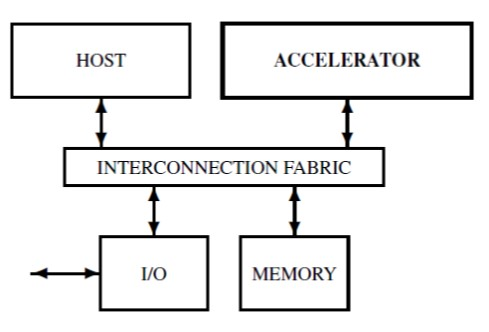# YPO2048

### Heterogenous computing

```complex segregated from intense
S: size of code expressed in number of line of code
T: time expressed in number of clock cycle
Complex computation: S ~ T
performed on HOST

Intense computation:   S << T
performed on ACCELERATOR

Programming model

HOST runs programs written using libraries such as Eigen, TensorFlow, XXX
ACCELERATOR is used to implement kernel libraries such as EigenKernel, TensorFlowKernel, XXXKernel
LibraryNameKernel: is the LibraryName library implemented for data structures with sizes limited by the parameters of ACCELERATOR
(number of cells, n, size of the local register files, m)
LibraryName is implemented in a high level language on HOST using LibraryNameKernel

Estimated performances for YPO2048
Technology node: 28 nm
fclock = 1 GHz
No. of cores: n = 2048 cells
Size of the register file in each cell: m = 1024 32-bit words
Die size: 	9.2 x 9.2 mm2
Power: 		12/14/18 Watt at 80/100/1200C
Integer arithmetic:
170 GOPS/Watt for 32-bit integers
340 GOPS/Watt for 16-bit integers
Floating point arithmetic:
63 GFLOPS/Watt for 32-bit floats
200 GFLOPS/Watt for 16-bit floats

Linear algebra
lines x col Matrix multiplied with a col - component vector,
where lines <=  m and col <= n is performed in

tmatrixVectorMult(lines,col) =  2 x lines +  log2 n is in O(lines)

YPOxxx performance is supra-linear:
for each component of the resulting vector a mono-core engine uses more than 2 clock cycles.

SHA-256
Nvidia s GeForce GTX TITAN X:
Technology node: 28 nm
Die size: 601 mm2
No. of cores: 3092
fclock  = 1 GHz
Power: 250 Watt
SHA performance: 15.688 MH/sec/Watt
Our YPO2048
SHA performance: 57.6 MH/sec/Watt (measured on cycle accurate simulator)
YPO2048 performance/Nvidia performance = 3.37

```